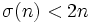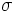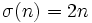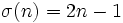# Deficient number

This article defines a property that can be evaluated for a natural number, i.e., every natural number either satisfies the property or does not satisfy the property.
View a complete list of properties of natural numbers

## Definition

A natural numberis termed a deficient number if, wheredenotes the divisor sum function. In other words,is greater than the sum of all its proper positive divisors.

## Relation with other properties

### Opposite properties

• Perfect number: This requires.
• Abundant number: This requires.

### Stronger properties

• Almost perfect number: A natural numbersuch that.